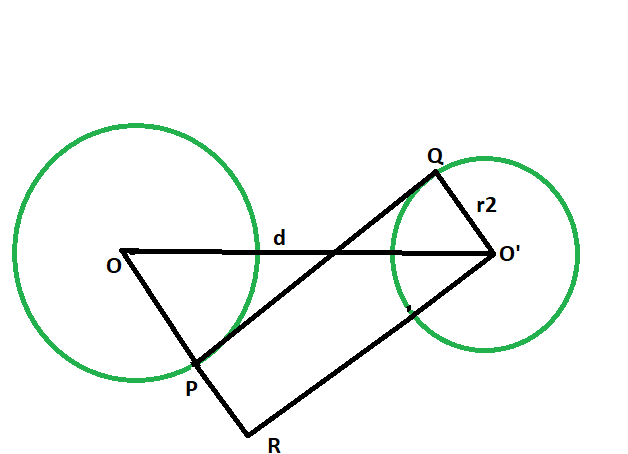Open in App
Not now

# Length of the transverse common tangent between the two non intersecting circles

• Last Updated : 03 Aug, 2022

Given two circles of given radii, having there centres a given distance apart, such that the circles don’t touch each other. The task is to find the length of the transverse common tangent between the circles.
Examples:

```Input: r1 = 4, r2 = 6, d = 12
Output: 6.63325

Input: r1 = 7, r2 = 9, d = 21
Output: 13.6015```Approach

1. Let the radii of the circles be r1 & r2 respectively.
2. Let the distance between the centers be d units.
3. Draw a line O’R parallel to PQ,
4. angle OPQ = angle RPQ = 90 deg
angle O’QP = 90 deg
{ line joining the center of the circle to the point of contact makes an angle of 90 degree with the tangent }

5. angle RPQ + angle O’QP = 180 deg
PR || O’Q
6. Since opposite sides are parallel and interior angles are 90, therefore O’PQR is a rectangle.
7. O’Q = RP = r2 and PQ = O’R
8. In triangle OO’R
angle ORO’ = 90 deg
By Pythagoras theorem
OR^2 + O’R^2 = OO’^2
O’R^2 = OO’^2 – OR^2
O’R^2 = d^2 – (r1+r2)^2
O’R^2 = √(d^2 – (r1+r2)^2)## C++

 `// C++ program to find the length` `// of the transverse common tangent` `// between two circles which` `// do not touch each other`   `#include ` `using` `namespace` `std;`   `// Function to find the length` `// of the transverse common tangent` `void` `lengthOfTangent(``double` `r1, ``double` `r2, ``double` `d)` `{`   `    ``cout << ``"The length of the transverse"` `         ``<< ``" common tangent is "` `         ``<< ``sqrt``(``pow``(d, 2) - ``pow``((r1 + r2), 2))` `         ``<< endl;` `}`   `// Driver code` `int` `main()` `{` `    ``double` `r1 = 4, r2 = 6, d = 12;` `    ``lengthOfTangent(r1, r2, d);` `    ``return` `0;` `}`

## Java

 `// Java program to find the length` `// of the transverse common tangent` `// between two circles which` `// do not touch each other` `class` `GFG {`   `    ``// Function to find the length` `    ``// of the transverse common tangent` `    ``static` `void` `lengthOfTangent(``double` `r1,` `                                ``double` `r2, ``double` `d)` `    ``{`   `        ``System.out.println(``"The length of the transverse"` `                           ``+ ``" common tangent is "` `                           ``+ Math.sqrt(Math.pow(d, ``2``)` `                                       ``- Math.pow((r1 + r2), ``2``)));` `    ``}`   `    ``// Driver code` `    ``public` `static` `void` `main(String args[])` `    ``{` `        ``double` `r1 = ``4``, r2 = ``6``, d = ``12``;` `        ``lengthOfTangent(r1, r2, d);` `    ``}` `}`   `// This code has been contributed by 29AjayKumar`

## Python3

 `# python 3 program to find the length` `# of the transverse common tangent` `# between two circles which` `# do not touch each other` `from` `math ``import` `sqrt, ``pow`   `# Function to find the length` `# of the transverse common tangent` `def` `lengthOfTangent(r1, r2, d):` `    ``print``(``"The length of the transverse"``,` `                     ``"common tangent is"``, ` `          ``'{0:.6g}'``.``format``(sqrt(``pow``(d, ``2``) ``-` `                                ``pow``((r1 ``+` `r2), ``2``))))`   `# Driver code` `if` `__name__ ``=``=` `'__main__'``:` `    ``r1 ``=` `4` `    ``r2 ``=` `6` `    ``d ``=` `12` `    ``lengthOfTangent(r1, r2, d)` `    `  `# This code is contributed by` `# Surendra_Gangwar`

## C#

 `// C# program to find the length` `// of the transverse common tangent` `// between two circles which` `// do not touch each other` `using` `System;`   `class` `GFG {` `    ``// Function to find the length` `    ``// of the transverse common tangent` `    ``static` `void` `lengthOfTangent(``double` `r1,` `                                ``double` `r2, ``double` `d)` `    ``{`   `        ``Console.WriteLine(``"The length of the transverse"` `                          ``+ ``" common tangent is "` `                          ``+ Math.Sqrt(Math.Pow(d, 2)` `                                      ``- Math.Pow((r1 + r2), 2)));` `    ``}`   `    ``// Driver code` `    ``static` `public` `void` `Main()` `    ``{` `        ``double` `r1 = 4, r2 = 6, d = 12;` `        ``lengthOfTangent(r1, r2, d);` `    ``}` `}`   `// This code has been contributed by ajit.`

## PHP

 ``

## Javascript

 ``

Output:

`The length of the transverse common tangent is 6.63325`

Time Complexity: O(logn) because using inbuilt sqrt and pow function

Auxiliary Space: O(1)

My Personal Notes arrow_drop_up
Related Articles